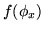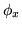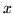Next: Non-spatial without Class Proportions Up: Modelling Previous: Spatial

## Continuous Weights Mixture Model

The discrete labels model does not allow the amount of spatial regularisation to be determined adaptively from the data. Therefore, in this section we will show how we can replace the discrete labels model with a new model that is an approximation to it. This new model does allow for the amount of spatial regularisation to be determined adaptively from the data.

The problem with the discrete labels model is that when we introduce spatial priors via the discrete MRF, we have an unknown functionas part of the prior (equation 6). It represents the effect which the MRF control parameter,, has on the normalising constant (also known as the partition function), and is required, up to a proportionality constant, to allow adaptive determination of the MRF control parameter,. The function,, can not be calculated analytically and computational evaluation is very difficult, requiring summation over all of the possible states of. As a result, the usual approach to such models (e.g. Zhang et al. (2001); Salli et al. (1999)) is to setto a fixed value based upon prior knowledge from other datasets of what makes a good value for. This means that the amount of spatial regularisation used by the MRF is non-adaptive.

To establish this approach we firstly introduce a vector of continuous class weights to replace the three discrete class models already described, whilst approximating the same posterior distribution. We then proceed to show how this allows adaptive determination of the amount of spatial regularisation in the spatial mixture models.

SubsectionsNext: Non-spatial without Class Proportions Up: Modelling Previous: Spatial